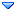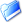GSI Forum
GSI Helmholtzzentrum für Schwerionenforschung

Home » Hades » Pluto » the simulation of ep to e delta(1910)+, delta(1910)+ to Sigma(1385)^0 K+
the simulation of ep to e delta(1910)+, delta(1910)+ to Sigma(1385)^0 K+ Wed, 27 April 2016 11:51Xinying Song Messages: 7Registered: January 2016 Location: Germany occasional visitor From: *ikp.kfa-juelich.de
Hi,

I want to simulate the process of ep-> e delta(1910)+, delta(1910)+-> Sigma(1385)^0 K+. My code is like this:

```
#include "TGraphAsymmErrors.h"
#include "TH1.h"
#include "TH2.h"
#include "TH3.h"
#include "TChain.h"
#include "TCanvas.h"
#include "TF1.h"
#include "TGraphAsymmErrors.h"
#include "TFile.h"
#include "TSystem.h"

#include "PParticle.h"
#include "PInclusiveModel.h"
#include "PReaction.h"
#include "PBeamSmearing.h"
#include "PAnyDistribution.h"
#include "plugins/scatter_mod/PScatterCrossSection.h"
#include "plugins/sigmatoLee/PSigmatoLee.h"

void tmp_YtoLambdaee() //int input, int events
{

int events = 100;
int input = 10;
double ebeam = 11.0;
bool xsection = 1;
gROOT->Reset();
PUtils::SetSeed(input*1000);
makeStaticData()->SetParticleTotalWidth("Sigma13850",0.0360);
makeStaticData()->SetParticleBaryon("Sigma13850",1);
makeStaticData()->SetParticleLMass("Sigma13850",1.115);
makeStaticData()->AddDecay("Sigma13850 --> Lambda + dilepton", "Sigma13850", "Lambda, dilepton", 1.0);
//makeStaticData()->AddDecay("dilepton --> e+ + e-", "dilepton", "e+, e-", 1.0);

makeStaticData()->SetParticleTotalWidth("Delta1910+",0.340);
makeStaticData()->SetParticleBaryon("Delta1910+",1);
makeStaticData()->SetParticleLMass("Delta1910+",1.89);
makeStaticData()->AddDecay("Delta1910+ --> Sigma13850 + K+", "Delta1910+", "Sigma13850, K+", 1.0);
//~~~~//makeStaticData()->AddDecay("Lambda --> p + pi- + dilepton", "Lambda", "p, pi-, dilepton",1.0);

PAnyDistribution* decay =
new PAnyDistribution("v_slope", "A function to add a new t-slope");
TH1F * cache  = new TH1F ("cache","v cache",400,0,11.0);
decay->AddEquation(cache,"beam = _parent->GetBeam(); beam->Boost(_parent) ; t1 = (beam - [e-,1])->E(); _x = t1;");

PScatterCrossSection *model = new PScatterCrossSection("mymodel","My cross section");

double M_P = 0.938272;   //Proton mass
double M_el = 0.000510999;   //Electron mass

//Define the range of the c.m. sampling
double En_beam = TMath::Sqrt(ebeam*ebeam + M_el*M_el);
double q= TMath::Sqrt((2.*En_beam*M_P)+(M_P*M_P) + (M_el*M_el));
double q_min = q - q/10000.;
double q_max = q + q/10000.;
//Now let set up the model

model->SetRange(q_min,q_max);
model->SetNpy(250);
model->SetNpx(250);  //Use this after SetNpy analysis

//Set up reaction as usual
char lund_file;

cout<<"#########################################################################"<<endl;
cout<<"################## Cross-Section Genertation is occuring ################"<<endl;
cout<<"#########################################################################"<<endl;
sprintf(lund_file,"%s","etaPXSection.lund");
/////////disribution of Sigma1385_0 to Lambda dileption
PSigmatoLee  *sigmadecaytoLee = new PSigmatoLee("sigma13850_to_e+_e-_lambda_matrix","sigma13850 to e+e-_Lambda Distribution");

PReaction *my_reaction = new PReaction("_P1 = 11.0","e-","p","e- Delta1910+ [Sigma13850 [dilepton Lambda] K+]","YtosigmaK",0,0,0,0);

my_reaction->Preheating(500);
my_reaction->Print();
my_reaction->Loop(events);
//

}
```

Here is the class written by myself which is put in /Pluto/pluto_v5.43/plugins/. I put it in the attachment and
There are errors like this when I run it,

it came out so many

Warning in <PDalitzDecay::dGdM>: Unknown decay

I look up this sentence in PDalitzDecay.cc, it may be because of there is no Dalitz decay of sigma13850->Lambda dilepton,though I defind this decay in plugins, So how to solve this problem?
•Attachment: PSigmatoLee.h
•Attachment: PSigmatoLee.cc

[Updated on: Wed, 27 April 2016 13:51]

Report message to a moderatorthe simulation of ep to e delta(1910)+, delta(1910)+ to Sigma(1385)^0 K+ By: Xinying Song on Wed, 27 April 2016 11:51Re: the simulation of ep to e delta(1910)+, delta(1910)+ to Sigma(1385)^0 K+ By: Ingo Froehlich on Fri, 29 April 2016 17:58Re: the simulation of ep to e delta(1910)+, delta(1910)+ to Sigma(1385)^0 K+ By: Xinying Song on Tue, 03 May 2016 13:03
 Previous Topic: differential cross section Next Topic: [SOLVED]generate rho0->pi+pi- but found the rho0 has no width
Goto Forum:

Current Time: Sat Jun 03 23:24:34 CEST 2023

Total time taken to generate the page: 0.00974 seconds